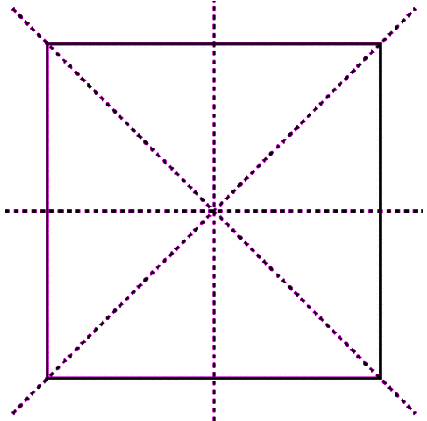Checkout JEE MAINS 2022 Question Paper Analysis : Checkout JEE MAINS 2022 Question Paper Analysis :

# Reflection And Symmetry

Nature gives us many examples of the relation between reflection and symmetry; the image of mountains and trees are reflected in the nearby water bodies, dew drops collected on leaves glimmer in the presence of sunlight etc. This concept was also used for decoration of forts and palaces, hundreds of years ago. For example, the roofs and walls of the mirror palace (Jaipur) were decorated with small pieces of mirror, which formed beautiful symmetrical patterns, thus adding to their aesthetic value.  This concept is now used for decorating jewelry shops and temples. Also, check Line Of Symmetry.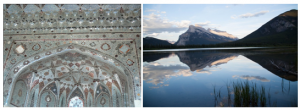In the process of reflection, the mirror image is nothing but the reflected duplication of the original object. Here, the resultant image is identical to the original object, but reversed in direction. For example, when the alphabet C is viewed in the mirror, the point to point distance of the original object and the reflected image is same with respect to the mirror, but the direction is reversed, as can be seen in the following image.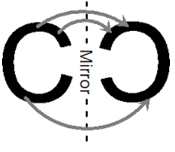## Reflection And Symmetry Applications

Kaleidoscope

Kaleidoscope is a device, typically cylindrical or triangular in shape, consisting of three or more mirrors. We put small colorful objects, such as beads and pieces of glass in the instrument. The light enters from one end and the viewers look through the other end. These mirrors act as reflectors and provide multiple line of symmetry to the patterns due to repeated reflections in the mirror.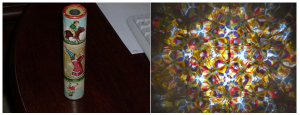## Mirror symmetry

Any image is said to have reflection or mirror symmetry if there is one or more than one lines such that, the first half is a mirror image of the second half. Reflection symmetry is also called mirror symmetry.  Some of our alphabets pose reflection symmetry, for example the alphabet A, H, W or M. If we place a mirror at the centre of any of these alphabets we get the reflection of the other half.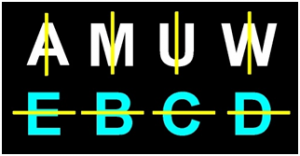## Symmetry for Shapes

Some of the geometric shapes have a line of symmetry. Some shapes have more than one line of symmetry. Let us see the symmetry and reflection with respect to the geometric shapes. Also, check Figures With Symmetry.

Rectangle

A rectangle has two lines of symmetry since it can be divided into two equal parts along width or length.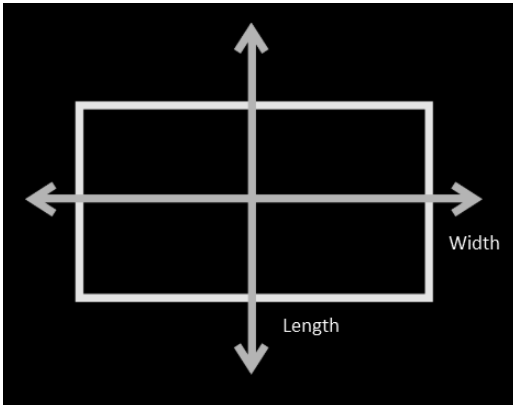Circle

A circle can be divided into two equal parts along its diameter. We know that a circle can have an infinite number of diameters and hence we can say that circles can have infinite lines of symmetry.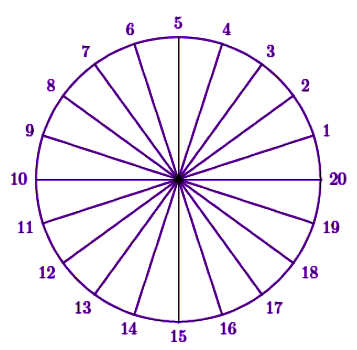As shown in the above diagram, we can draw multiple diameters in a circle.

Square

In the same way, a square can be divided into two equal parts along its diagonal and side (vertically, horizontally). Thus a square has four lines of symmetry.